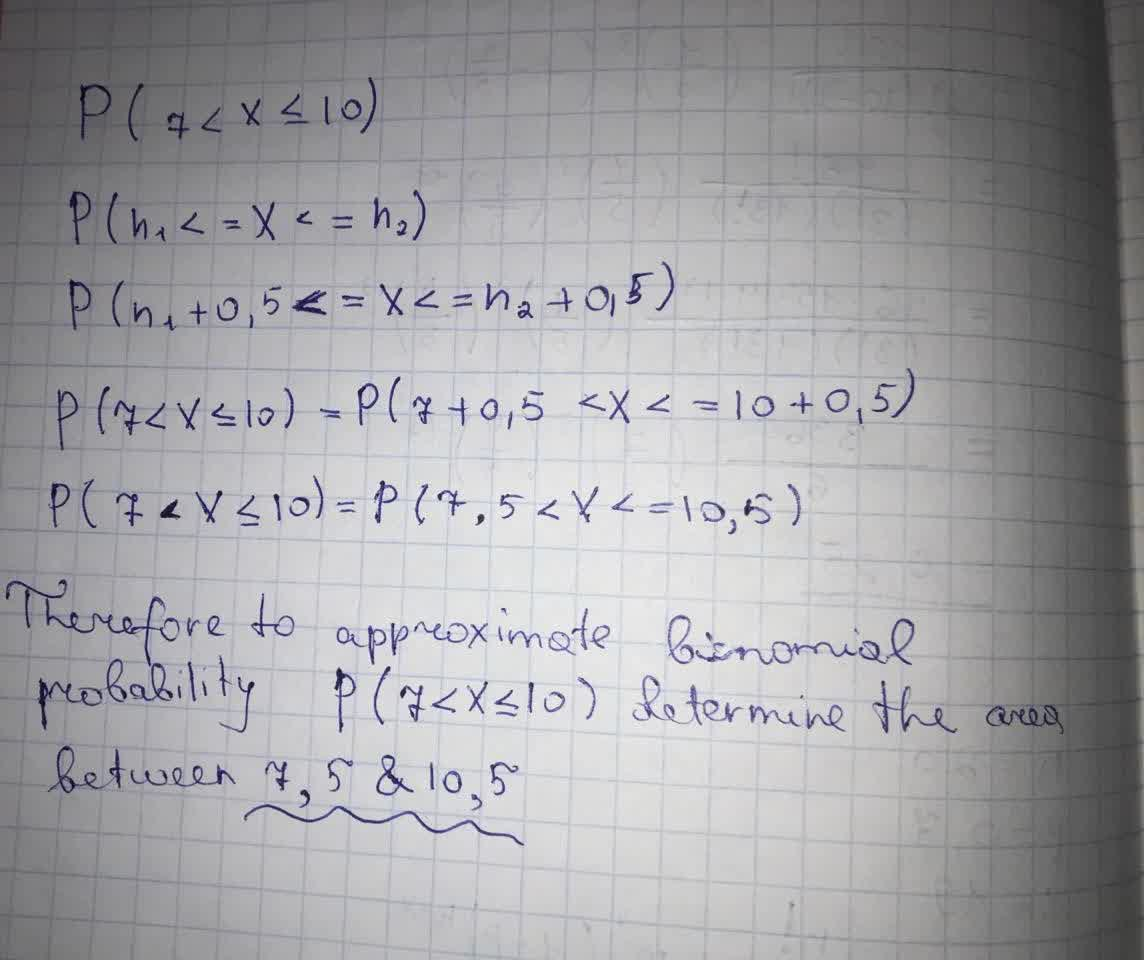Khaleesi Herbert

2021-09-08

X denotes a binomial random variable with parameters n and p. For each exercise, indicate which area under the appropriate normal curve would be determined to approximate the specified binomial probability. $P\left(7Viktor Wiley